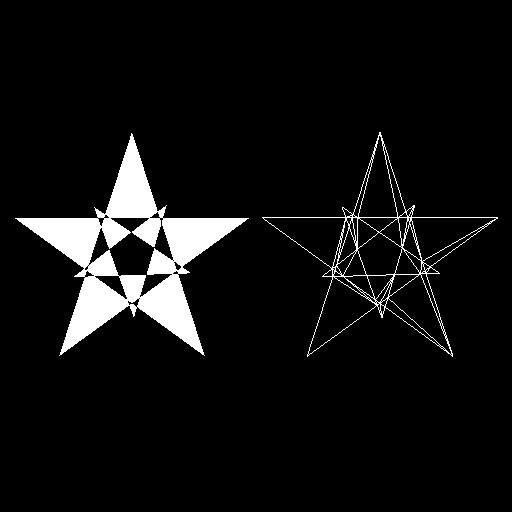Visualization Library 2.1.0 A lightweight C++ OpenGL middleware for 2D/3D graphics VL     Star     Watch     Fork     Issue [Download] [Tutorials] [All Classes] [Grouped Classes]
Polygon Tessellation Tutorial

In this tutorial you will learn how to use the vl::Tessellator class to tessellate a concave or self intersecting polygon into a set of triangles that can be rendered with Visualization Library.[From `App_Tessellator.cpp`]

class App_Tessellator: public BaseDemo
{
public:
App_Tessellator() {}
void initEvent()
{
// Basic initialization
vl::Log::notify(appletInfo());
// Filled effect
// Wireframe effect
vl::ref<vl::Effect> wireframe_fx = new vl::Effect;
mStar1 = sceneManager()->tree()->addActor( new vl::Actor(NULL, filled_fx.get(), new vl::Transform) );
mStar2 = sceneManager()->tree()->addActor( new vl::Actor(NULL, wireframe_fx.get(), new vl::Transform) );
}
{
// Animation: compute rotation matrix to rotate the small star, 45�/sec rotation.
// Filled star on the left, wireframe star on the right
mStar1->transform()->setLocalMatrix( vl::mat4::getTranslation(-4,0,0) );
mStar2->transform()->setLocalMatrix( vl::mat4::getTranslation(+4,0,0) );
// Concave and self-intersecting polygons cannot be directly rendered by OpenGL, for this
// reason in order to render them we have to tessellate them first, i.e. we have to decompose
// them into a set of triangles using the Tessellator class.
// The Tessellator class takes as input a set of contours defining a complex polygon and
// outputs a series of triangles that can be rendered by OpenGL and Visualization Library
tess.setTessNormal(vl::fvec3(0,0,1)); // default is vl::fvec3(0,0,0)
tess.setBoundaryOnly(false); // default
tess.setTolerance(0.0); // default
// Outline #1 - generate 5-points star (small)
int size = 2;
tess.contours().push_back(5);
for(int i=0; i<5; ++i)
{
float t = (float)i/5.0f*vl::fPi*2.0f*2.0f + vl::fPi/2.0f;
// Fill the first contour
tess.contourVerts().push_back( m * vl::dvec3(cos(t)*size,sin(t)*size,0.0f) );
}
// Outline #2 - generate 5-points star (big)
size = 4;
tess.contours().push_back(5);
for(int i=0; i<5; ++i)
{
float t = (float)i/5.0f*vl::fPi*2.0f*2.0f + vl::fPi/2.0f;
// Fill the second contour
tess.contourVerts().push_back( vl::dvec3(cos(t)*size,sin(t)*size,0.0f) );
}
// Tessellate the two contours into a single polygon
tess.setTessellateIntoSinglePolygon(true); // default
tess.tessellate();
/*
You can also tessellate each contour separately selecting setTessellateIntoSinglePolygon(false).
This way each contour will be processed separately and will generate its own set of triangles, this
is useful when you want to tessellate a large number of polygons with a single tessellate() call.
*/
// Create a new Geometry and vertex array with the tessellated triangles
tess_poly->setVertexArray(vert_array.get());
// Fill the vertex array with the tessellated triangles
vert_array->initFrom(tess.tessellatedTris());